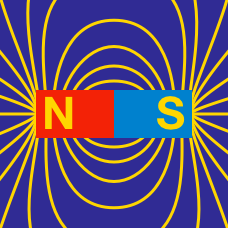Electricity and Magnetism

# Magnetic flux and Faraday's law (qualitative)

Suppose that when you move a magnet through a $40$-turn coil at a certain speed, a voltage of $10\text{ V}$ is induced across the coil. If you move the same magnet through a $80$-turn coil at the same speed, what voltage will be induced across the coil, assuming both coils are closely packed?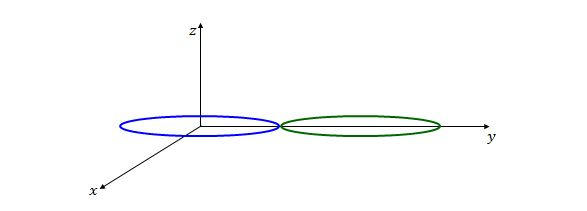Two circular loops lie in the $xy$-plane and the $z$-axis passes through the center of the left loop, as shown in the above figure. By switching on, a counterclockwise current start to flow in the left loop, as viewed from a point on the positive $z$-axis. Then which of the following statements is true of the right loop at that moment?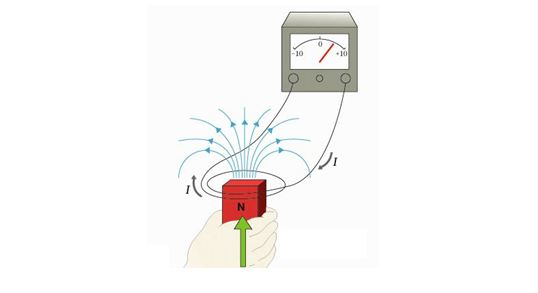According to Faraday's law, an ammeter resists a current in the wire loop when the magnet is moving with respect to the loop. Which of the following can induce an emf?

(a) Change the magnitude $B$ of the magnetic field within the loop.
(b) Change the total area of the loop that lies within the magnetic field.
(c) Change the angle between the direction of the magnetic field $\vec{B}$ and the plane of the loop.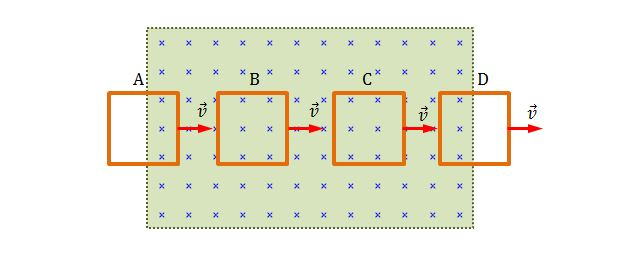A wire loop is moving with constant velocity $v$ through a uniform magnetic field, as shown in the above diagram. This field may be produced, for example, by a large electromagnet. The dotted rectangle shows the assumed limits of the magnetic field; the fringing of the field at its edges is neglected. The magnetic field is directed into the plane of the screen. Choose the moment at which the current $i$ is induced in the wire loop.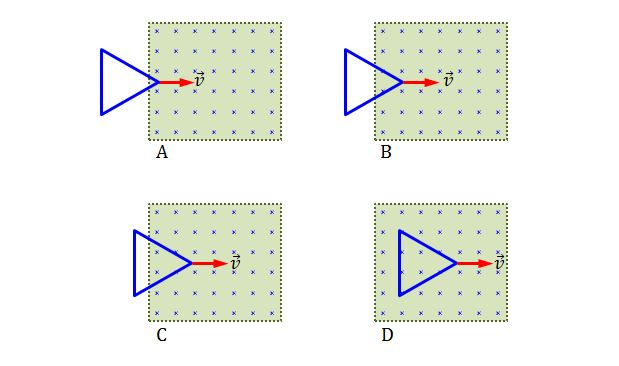In diagram A above, an equilateral triangle loop is just entering, at time $t_A=0,$ a region of uniform magnetic field. In diagram B, C and D at some later times $t_D > t_C > t_B > t_A,$ the triangle loop has moved distances $x_1,$ $x_2$ and $x_3,$ respectively, into the magnetic field. If the triangle loop is moving with constant velocity $v,$ what is the appropriate order of the magnitudes of the induced current $i$ in the triangle loop?

×